###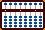Soroban and Shifting the Unit Rod - Multiplication & Division

I've always been fascinated by decimal numbers. In earlier tutorials I've discussed at length how I deal with decimal numbers when solving problems of multiplication and division. Here's yet another method that's so simple I find I'm using it almost exclusively. While this method is similar to predetermining the unit rod, the biggest difference is the decimal place is determined after completing the problem.

Although this is a soroban technique and I'm using Kojima's methods for both multiplication and division these examples could also be solved using a 2/5 bead suan pan.

Using these techniques to find the correct decimal answer is remarkably straightforward. Solve the problem and simply count either the whole numbers or trailing zeros in the the multiplier or divisor. Then shift the unit rod left or right accordingly. It's that easy. Here's how it works.

##### In division always start the count beginning one rod to the left of the unit rod (in this case rod E)### Multiplication And Shifting the Unit Rod

Example 1:   67 x 0.023 = 1.541

Choose rod F to be the unit rod and set the problem remembering that the multiplier is 0.023 on rods AB. (Fig. 1)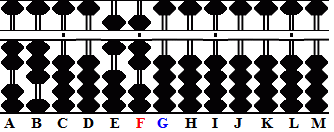(Fig. 1)

Step 1: Using Kojima's multiplication techniques solve the problem leaving 1541.

Step 2 and the answer: Choose the new unit rod. The multiplier has one trailing zero. Starting on rod G (one rod to the right of the unit rod) count one to the left. Rod F remains the unit rod, therefore the answer to 67 x 0.023 = 1.541 (Fig. 2)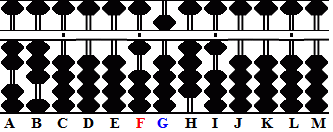(Fig.2)

Example 2:   0.067 x 2.3 = 0.1541

Choose rod C to be the unit rod and set the problem remembering that the multiplier is 2.3 on rods AB.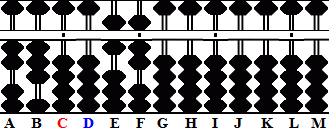(Fig. 3)

Step 1: Using Kojima's multiplication techniques solve the problem leaving 1541.

Step 2 and the answer: Choose the new unit rod. The multiplier has one whole number. Starting on rod D (one rod to the right of the unit rod) count one rod to the right. The new unit rod is rod E therefore the answer to  0.067 * 2.3 = 0.1541 (Fig. 4)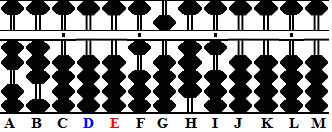(Fig.4)

Example 3:   67,000 x 0.23 = 15410

Choose rod I to be the unit rod and set the problem remembering that the multiplier is 0.23 on rods AB. (Fig. 5)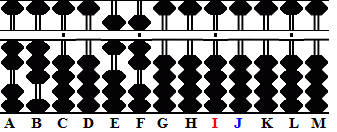(Fig. 5)

Step 1: Using Kojima's multiplication techniques solve the problem leaving 1541.

Step 2 and the answer: Choose the new unit rod. The multiplier has neither whole numbers nor trailing zeros.  Starting on rod J (one rod to the right of the unit rod) count neither right nor left. The new unit rod is rod J therefore the answer to  0.23 x 67,000 = 15410 (Fig. 6)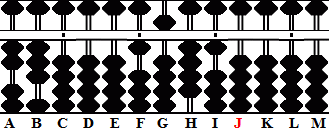(Fig. 6)

### Division And Shifting the Unit Rod

Example 1: 1541 ÷ 23 = 67

Choose rod I to be the unit rod and set the problem remembering that the divisor is 23 on rods AB. (Fig. 7)(Fig. 7)

Step 1: Using Kojima's division techniques solve the problem leaving 67.

Step 2 and the answer: Choose the new unit rod. The divisor has two whole numbers. Starting on rod H (one rod to the left of the unit rod) count two to the left. The new unit rod is rod F therefore the answer to 1541 ÷ 23 = 67 (Fig. 8)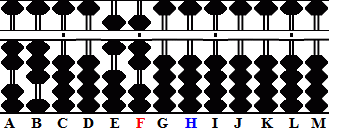(Fig. 8)

Example 2:
15.41 ÷ 0.0023 = 6700

Choose rod F to be the unit rod and set the problem remembering that the divisor is 0.0023 on rods AB. (Fig. 9)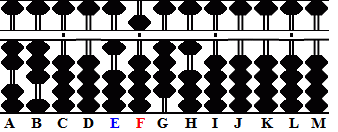(Fig. 9)

Step 1: Using Kojima's division techniques solve the problem leaving 67.

Step 2 and the answer: Choose the new unit rod. The divisor has two trailing zeros. Starting on rod E (one rod to the left of the unit rod) count two to the right. The new unit rod is rod G therefore the answer to 15.41 ÷  0.0023 = 6700 (Fig. 10)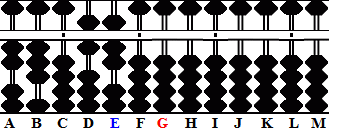(Fig. 10)

Example 3: 1.541 ÷ 0.23 = 6.7

Choose rod F to be the unit rod and set the problem remembering that the divisor is 0.23 on rods AB. (Fig. 11)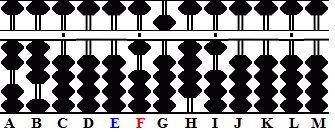(Fig. 11)

Step 1: Using Kojima's division techniques solve the problem leaving 67.

Step 2 and the answer: Choose the new unit rod. The divisor  has neither whole numbers nor trailing zeros.  Starting on rod E (one rod to the left of the unit rod) count neither right nor left. The new unit rod is rod E therefore the answer to 1.541 ÷ 0.23 = 6.7 (Fig. 12)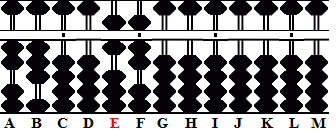(Fig. 12)

##### Print Shifting the Unit Rod
 Two alternative methods  Locating the Decimal 1 & Locating the Decimal 2